# Lesson1 Different Types in Javascript

It’s going to be first lesson on JavaScript and in this lesson, we are finally learning about JavaScript and its syntax, in the first place
we are going to start with its basic built in primitive data types. We have a few objectives here:

### Objectives

1. Introduce the 5 primitive data types.
2. Work with numbers and numeric operators.
3. Work with strings and common string methods.

## 5 Primitive Data types

So one of the big ideas at the core of every programming language is the language ability to differentiate between different categories of data.
For instance, a language could differentiate between a number and a word or it could differentiate between whole number and fractional number or a positive number and or a negative number and it varies from language to language to language.
In JavaScript there are five low level basic types of data and we are going to do a quick overview of the first and then we are going to jump into the detail.

## Number Type

So the first one is number and we have three examples here.
A whole number that is ‘7’ for a fractional, a decimal number 10.5 and a negative number 20.
I chose these three examples because I wanted to show you that JavaScript doesn’t care if a number is in fraction, negative and whole, JavaScript treated all as the numbers.
So other languages do differentiate but JavaScript doesn’t.
So again numbers are just a category for whole numbers fractional numbers and negative numbers.
So let’s dive a little bit deeper into JavaScript numbers type, go and open JavaScript console, simply open your Google chrome browser and view Page source.
You can do this with any site.
I am going to write so codes here.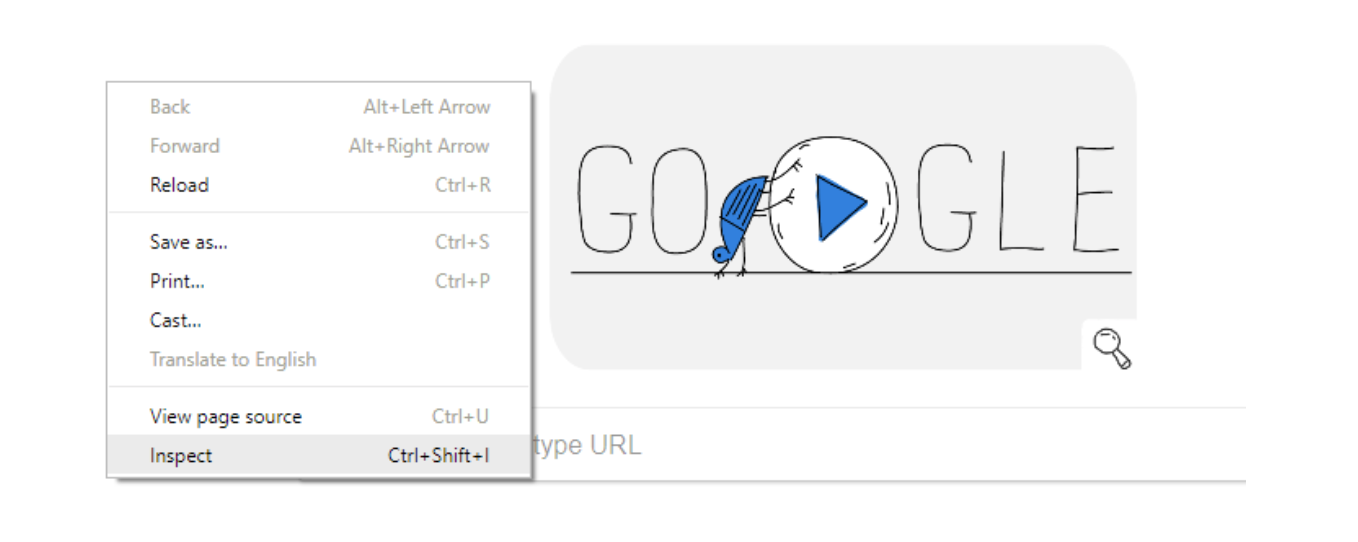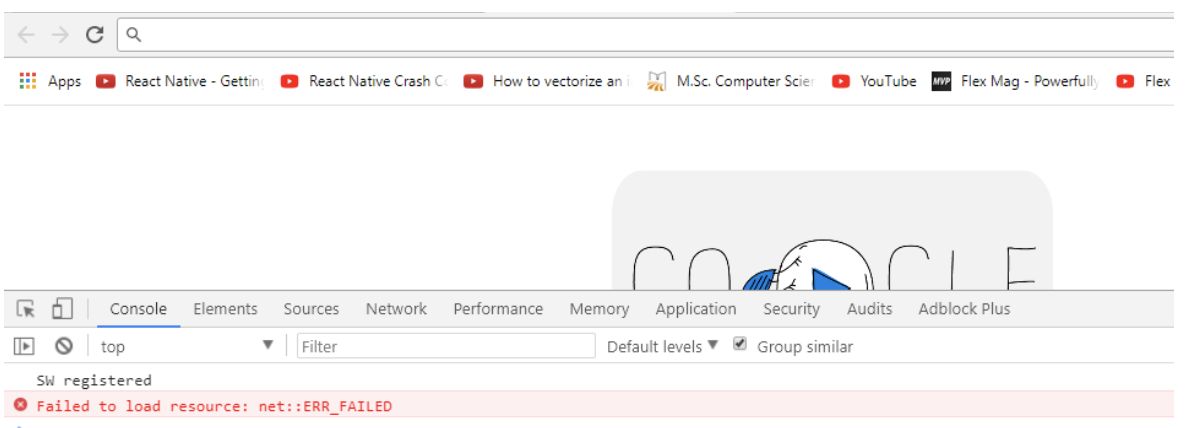If you write any number then it will get back to you exactly like you enter.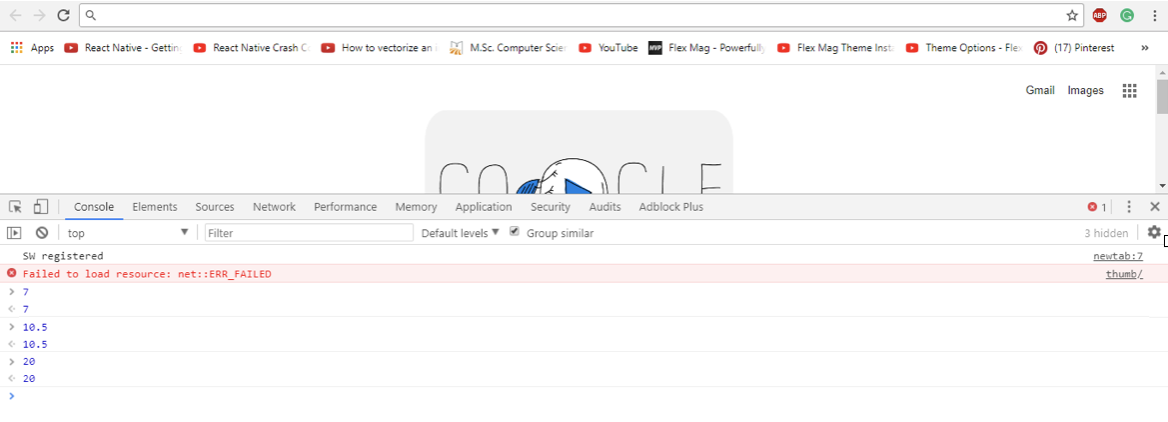You can also do mathematical solutions and JS follows the mathematical rules.
So performing things like addition, Multiplication, division and subtraction are really easy and the way that those work write some similar expression and hit enter.
Here is an example of numbers below.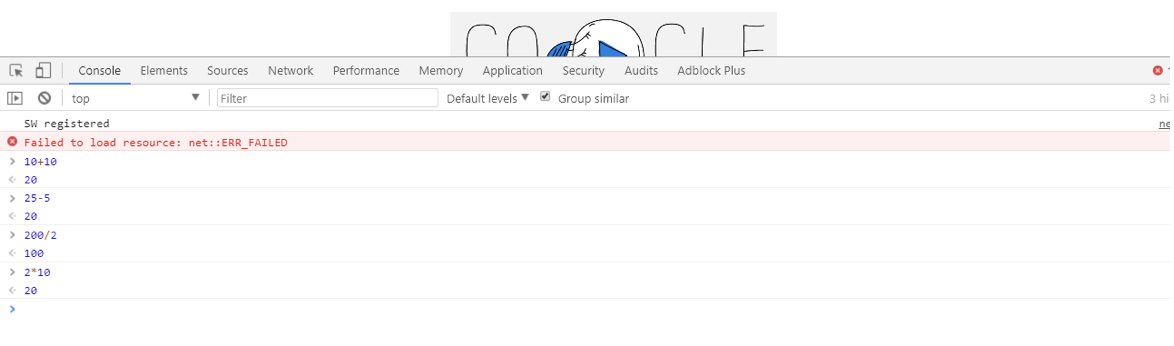You can see in the output that we have performed different task like multiplication, division, subtraction and addition by straight typing the mathematical equation in a Chrome Developer Console.

## Modulo Operator / Remainder Operator

Another chrome’s operator which is quiet unfamiliar to those who has no background knowledge of programming.
Remainder operator uses a percentage sign (%).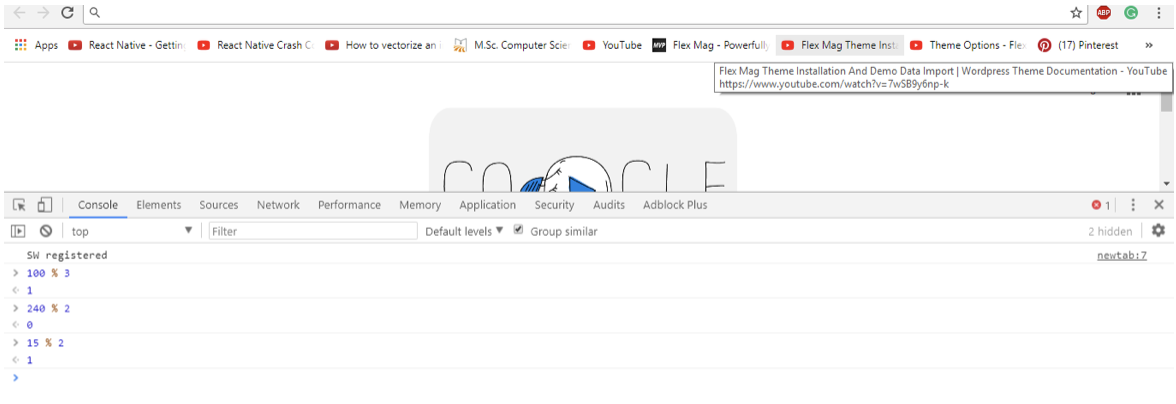If we write 100 modulo (%) 3 = Then it will take 3 and divided it into 100 as many times as it goes in as a whole number.
So that would be 33 times and then it will take the remainder that is 1.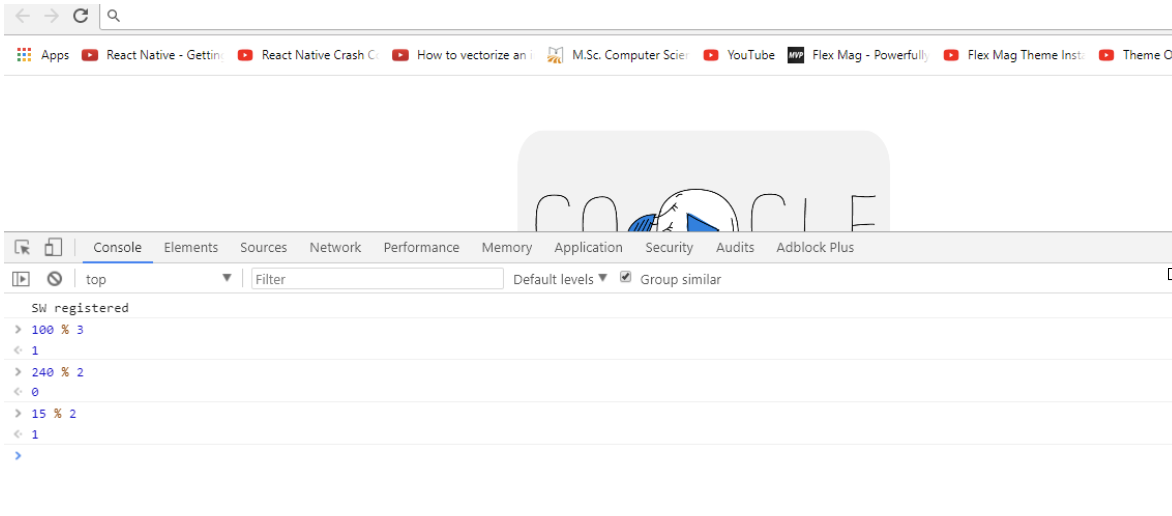## String Type

The next category that we have is called strings. They are basically a text. The important thing is that they are inside of quotes. Here we have two examples, so we have the word “Hellow Geeks” which is inside of quotes. This is one string even though it is consist of multiple words. Here is an example of string below: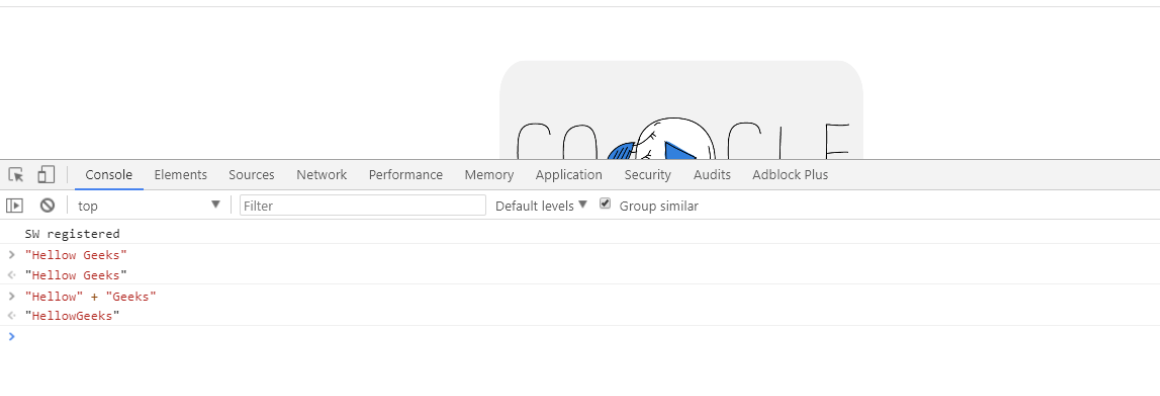Same thing happens with numbers in string, every number inside of quotes considered a string in JavaScript.
You can see here we have a number inside of quotes “500”, it is considered string to JavaScript.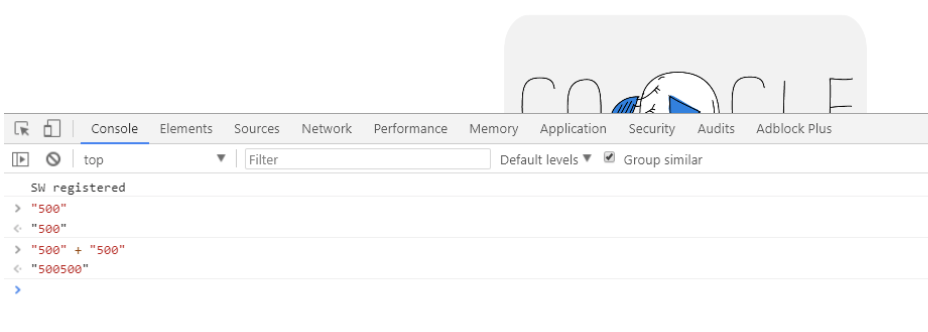Quotes can be of double and single type.
So just as an example If we type “Hello Geeks!” and hit enter, it just spits the value back as it is.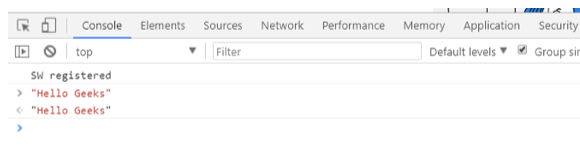Same rule applies with the single quote: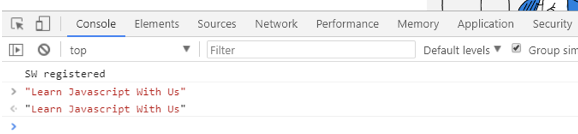In this lesson we have learned about basic of JavaScript and we have disused basic operation of JavaScript like Addition, subtraction, division and multiplication and we have also discussed strings in Javascript.